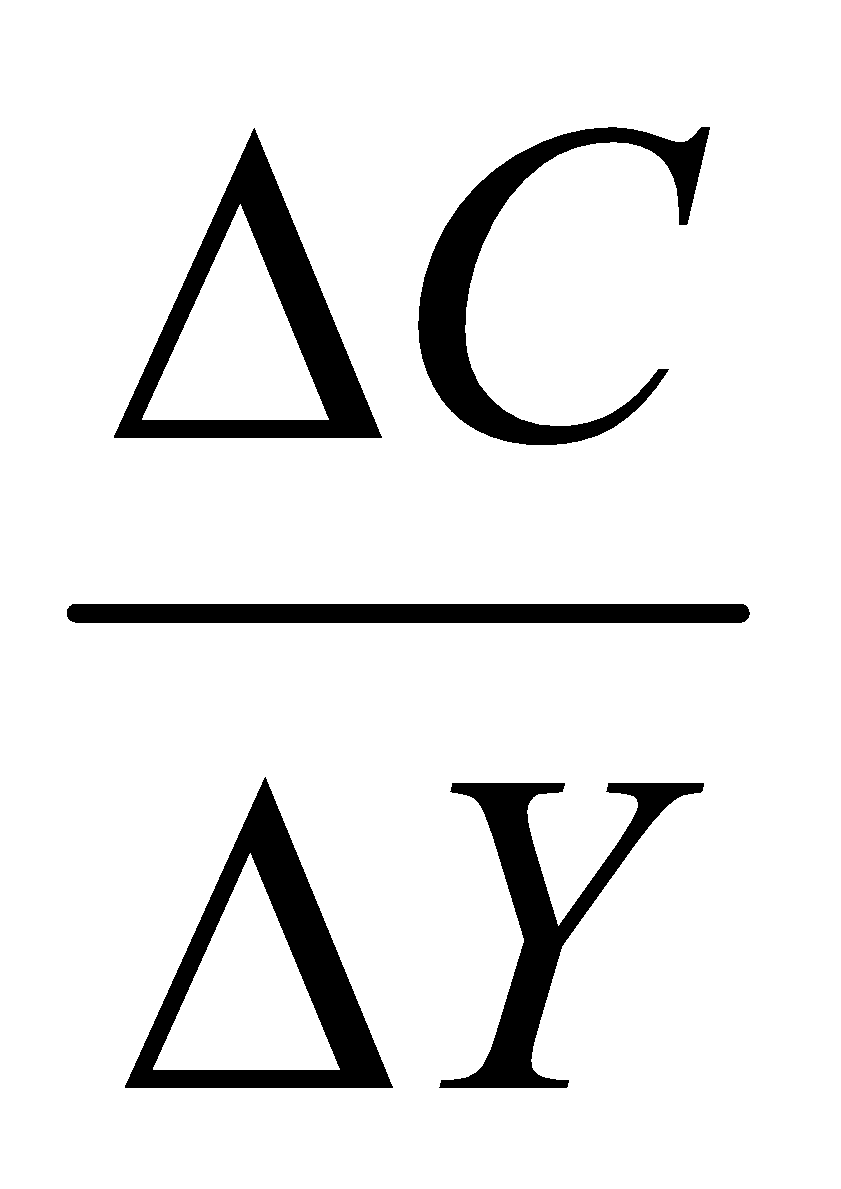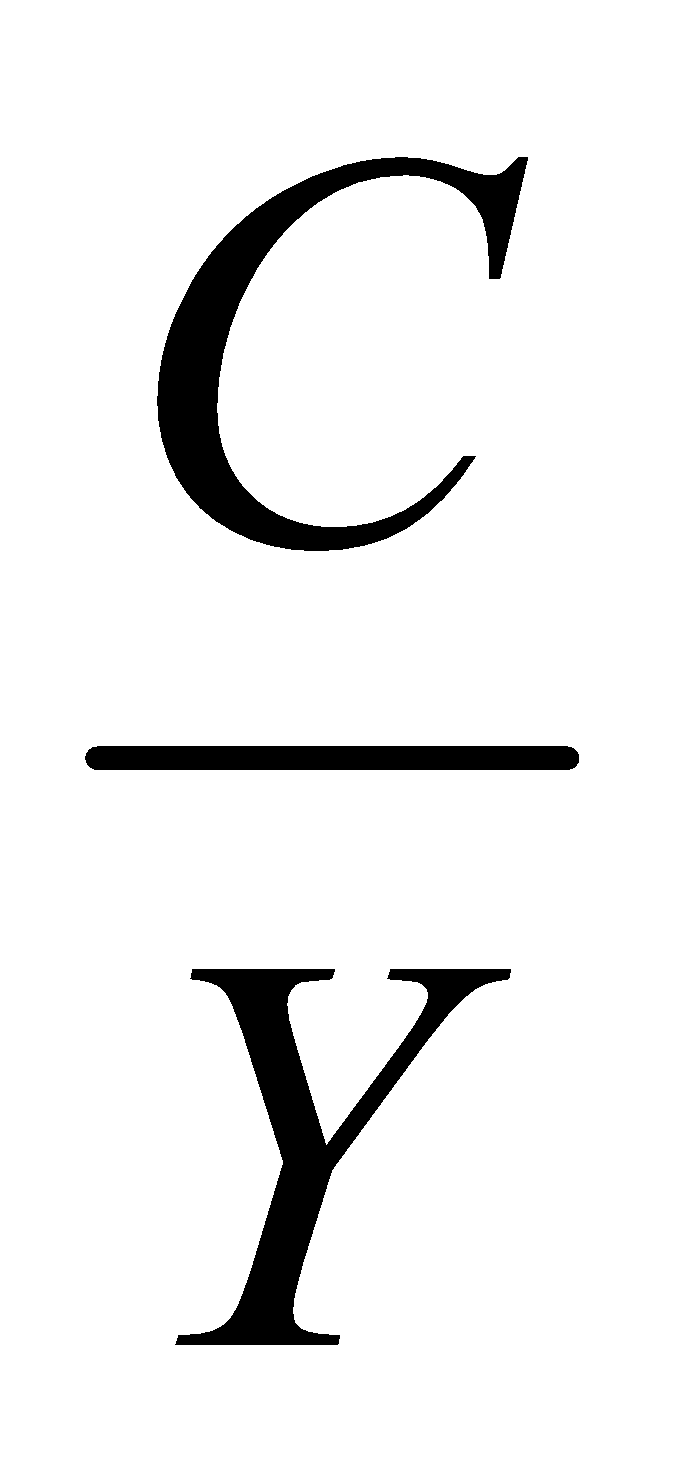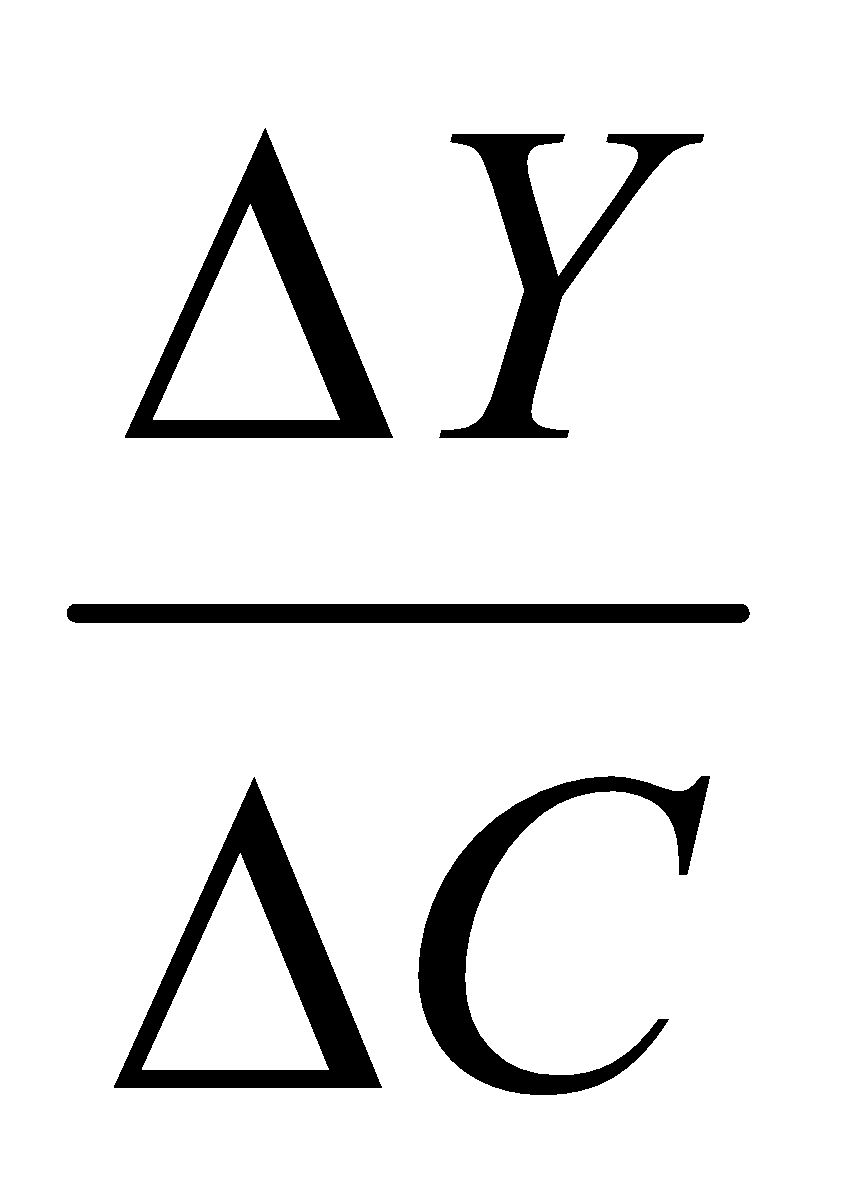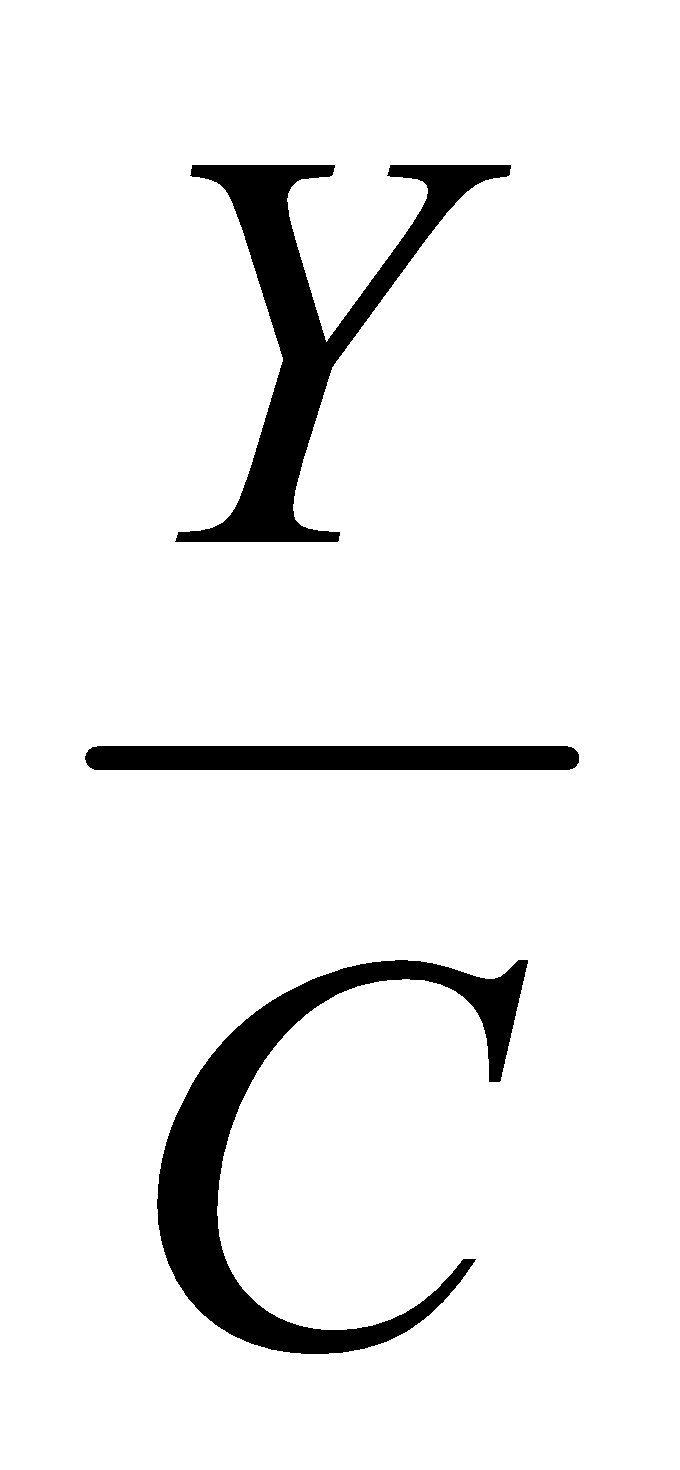## Friday, January 04, 20192014
(May)
ECONOMICS
(General)
Course: 201
(Macroeconomics)
Full Marks: 80
Pass Marks: 32
Time: 3 hours
The figures in the margin indicate full marks for the questions

1. National income is a
1. Flow.
2. Stock.
3. Fund.
4. Capital.
1. Average propensity to consume is
1.2.3.4.1. The bank having the legal power of issuing currency notes is
1. Commercial Bank.
2. Central Cooperative Bank.
3. Central Bank.
4. Regional Rural Bank.
1. During deflation, price level
1. Falls.
2. Rises.
3. Remains constant.
1. Who propounded the purchasing power parity theory?
2. In India, liberalization started in
1. 1951.
2. 1981.
3. 1991.
4. 1947.
1. The permanent income hypothesis was given by
1. Keynes.
2. Fisher.
3. Friedman.
4. Duessenberry.
1. Which of the following is not a method of measuring gross national product?
1. Expenditure method.
2. Income method.
4. None of the above.
2. Write short notes on any four (within 150 words each): 4x4=16
1. Main issues of macroeconomics.
2. Index numbers.
3. Classical theory of employment.
4. Open-market operations.
Answer the following questions (within 500 words each):
3. (a) What is meant by national income? Explain different methods of computing national income. How national income statistics help in formulating plan in a country? 2+6+4=12
Or
(b) Define the following concepts: 2x6=12
1. Net national product.
2. National income.
3. Real gross domestic product.
4. Personal income.
5. Disposable personal income.
6. Per capita income.
4. (a) Explain critically the cash transactions approach to the quantity theory of money. 11
Or
(b) Define money. What do you mean by legal tender money and non-legal tender money? Explain briefly the modern concept of demand for money.
5. (a) Explain the role of commercial bank in economic development. 11
Or
(b) Explain the functions of central bank. Name the central bank of India. 10+1=11
6. (a) Explain the meaning of average propensity to consume and marginal propensity to consume. Discuss the subjective factors affecting consumption function. 5+6=11
Or
(b) Analyze the Keynesian theory of employment. How far is the theory relevant in the developing economy?  9+2=11
7. (a) Explain with example the comparative advantages theory of international trade. What are the drawbacks of this theory? 8+3=11
Or
(b) What are the major objectives of International Monetary Fund (IMF)? Discuss the functions of IMF.     3+8=11

***